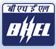# Placement Papers - BHELBHEL PAPER - 10 AUG 2008 - SECUNDERABAD
Posted by :
Shravz
(44)
Hi Friends

Today I have taken BHEL exam. I would like to give u the details of the written test. It comprises of 2 sections Technical (120) and Aptitude (120), total 240 (Test duration 150 minutes). Here I will post the questions as far as I remember. I am from mechanical stream. Paper is easy and many are direct questions, only factor concerned is the time.

SECTION-I--TECHNICAL

1. Critical load acting axially on a long column
Ans: (pi)2*E*I/L2

2. Relation between moment of area and radius of gyration
Ans: I=AK2

3. Cup shaped components are produced using which operation
Ans: drawing

4. A sheet of Al alloy having 2mm thickness is welded using which process
Ans: TIG

5. Two gears having teeth 18 and 40 and center distance 56.. what is the module?

6. In thick cylinders, which of the following is true[*S stands for sigma*]
(a) St>Sr (b) St=Sr (c)St

7. In a plane stress condition, if the centre of Mohr\'s circle coincides with the origin then which is true.. ...the relation between Sx and Sy is asked

8. Lame\'s theorem...F1/sinA=F2/sinB=F3/sinC

9. Young\'s modulus value for steel=200Gpa

10. Thermal conductivity of silver=400w/m0K

11. Fouling factor formula....F.F =1/Udirty-1/Uclean

12. Relation between notch sensitivity factor and theoretical stress concentration factor

13. Effectiveness of heat exchanger definition

14. Reynolds\'s number formula

15. Clinical thermometer is which order system ...0,1,2, or 3

16. Piezometric transducer is used to find which signals-static or... dynamic

17. Peclet number formula=Re*Pr

19. Which of the following is not related to CI engine
(a) Fuel pump (b) carburetor (c).. (d) none

20. Two connected in series having stiffness\'s K1, K2
Kequivalent=K1*K2/K1+ K2

21. Magnification factor at resonance, MF=1/2zeta

22. Coroili\'s component value, =2vw

23. Hooks law holds good upto
(a)elastic point (b)UYP(c)LYP(d)Failure

24. Meshing of two gears fail if
(a) materials are different(b)

25. A beam whose one end is fixed and other is free is
(a)cantilever (b)ss(c)Udl(d)none

26. Deflection at the free end of cantilever =PL3/3EI

27. Shear stress, twisting moment given ,find diamaeter...

28. Formula for polar moment of inertia of a hollow shaft

29. Formula for logarithmic decrement

30. A problem based on the above formula

31. Two question on transmissibility (W/Wn ratio)

32. Question from vibration..mass, k given.. cal frequency

33. Two more questions, one each from riveted joints and cotterjoint

34. 1BHN=N/m2

35. Temperature limits, given to find Carnot\'s efficiency

36. Metacentric height is the distance between Metacentre and ----
Ans: Centre of gravity

37. Force and displacement vector given to find out the work done

38. Rota meter is used to find
(a)flow(b)velocity(c)pressure(d)density